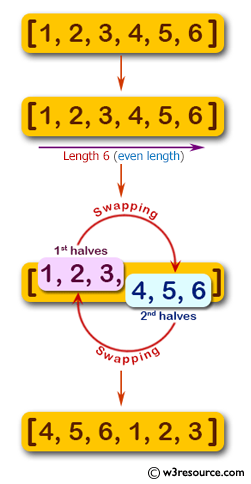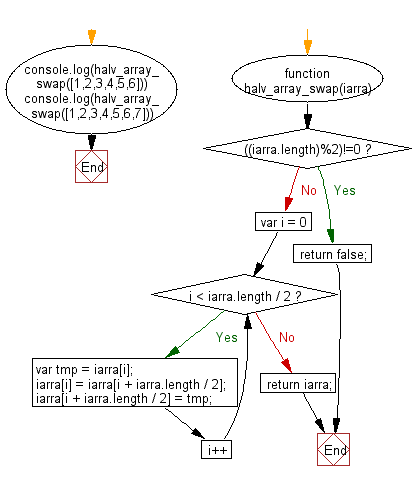# JavaScript: Swap two halves of a given array of integers of even length

## JavaScript Basic: Exercise-148 with Solution

Write a JavaScript program to swap two halves of a given array of integers of even length.

Pictorial Presentation:Sample Solution:

HTML Code:

``````<!DOCTYPE html>
<html>
<meta charset="utf-8">
<meta name="viewport" content="width=device-width">
<title>Swap two halves of a given array of integers of even length.</title>
<body>

</body>
</html>
```
```

JavaScript Code:

``````function halv_array_swap(iarra) {
if (((iarra.length)%2)!=0)
{
return false;
}

for (var i = 0; i < iarra.length / 2; i++) {
var tmp = iarra[i];
iarra[i] = iarra[i + iarra.length / 2];
iarra[i + iarra.length / 2] = tmp;
}
return iarra;
}

console.log(halv_array_swap([1,2,3,4,5,6]))
console.log(halv_array_swap([1,2,3,4,5,6,7]))
``````

Sample Output:

```[4,5,6,1,2,3]
false
```

Flowchart:ES6 Version:

``````function halv_array_swap(iarra) {
if (((iarra.length)%2)!=0)
{
return false;
}

for (let i = 0; i < iarra.length / 2; i++) {
const tmp = iarra[i];
iarra[i] = iarra[i + iarra.length / 2];
iarra[i + iarra.length / 2] = tmp;
}
return iarra;
}

console.log(halv_array_swap([1,2,3,4,5,6]))
console.log(halv_array_swap([1,2,3,4,5,6,7]))
``````

Live Demo:

See the Pen javascript-basic-exercise-148 by w3resource (@w3resource) on CodePen.

Improve this sample solution and post your code through Disqus

What is the difficulty level of this exercise?

Test your Programming skills with w3resource's quiz.

﻿

## JavaScript: Tips of the Day

Checks if a string is an anagram of another string (case-insensitive, ignores spaces, punctuation and special characters)

Example:

```const isAnagram = (str1, str2) => {
const normalize = str =>
str
.toLowerCase()
.replace(/[^a-z0-9]/gi, '')
.split('')
.sort()
.join('');
return normalize(str1) === normalize(str2);
};
console.log(isAnagram('iceman', 'cinema')); // true
```

Output:

```true
```